CAT  >  Test: XAT Quant 2018

# Test: XAT Quant 2018 - CAT

Test Description

## 27 Questions MCQ Test - Test: XAT Quant 2018

Test: XAT Quant 2018 for CAT 2023 is part of CAT preparation. The Test: XAT Quant 2018 questions and answers have been prepared according to the CAT exam syllabus.The Test: XAT Quant 2018 MCQs are made for CAT 2023 Exam. Find important definitions, questions, notes, meanings, examples, exercises, MCQs and online tests for Test: XAT Quant 2018 below.
Solutions of Test: XAT Quant 2018 questions in English are available as part of our course for CAT & Test: XAT Quant 2018 solutions in Hindi for CAT course. Download more important topics, notes, lectures and mock test series for CAT Exam by signing up for free. Attempt Test: XAT Quant 2018 | 27 questions in 40 minutes | Mock test for CAT preparation | Free important questions MCQ to study for CAT Exam | Download free PDF with solutions
 1 Crore+ students have signed up on EduRev. Have you?
Test: XAT Quant 2018 - Question 1

### Find the value of the expression: 10 + 103 + 106 + 109

Detailed Solution for Test: XAT Quant 2018 - Question 1
10 + 103 + 106 + 109 = 10 + 1000 + 1000000 + 1000000000

=1001001010

Therefore, option d is the right answer.

Test: XAT Quant 2018 - Question 2

### Abdul, Bimal, Charlie and Dilbar can finish a task in 10, 12, 15 and 18 days respectively. They can either choose to work or remain absent on a particular day. If 50 percent of the total work gets completed after 3 days, then, which of the following options is possible?

Detailed Solution for Test: XAT Quant 2018 - Question 2
Let us assume the amount of work to be finished = LCM of {10, 12, 15, 18} = 180 units.

The amount of work which Abdul can complete in a day = 180/10 = 18 units.

The amount of work which Bimal can complete in a day = 180/12 = 15 units.

The amount of work which Charlie can complete in a day = 180/15 = 12 units.

The amount of work which Dilbar can complete in a day = 180/18 = 10 units.

It is given that 50 percent of the total work gets completed after 3 days. Therefore, we can say that 90 units of work was completed in 3 days.

Let us check options.

Option (a): Each of them worked for exactly 2 days.

In this case amount of work completed = 2*(10+15+12+18) = 110 units.

Option (b): Bimal and Dilbar worked for 1 day each, Charlie worked for 2 days and Abdul worked for all 3 days.

In this case amount of work completed = 1 *(10+15) +2*(12) +3*(18) = 103 units.

Option (c): Abdul and Charlie worked for 2 days each, Dilbar worked for 1 day and Bimal worked for all 3 days.

In this case amount of work completed = 1 *(10) +3*(15) +2*(18+12) = 115 units.

Option (d): Abdul and Dilbar worked for 2 days each, Charlie worked for 1 day and Bimal worked for all 3 days.

In this case amount of work completed = 1 *(12) +3*(15) +2*(18+10) = 113 units.

Option (e): Abdul and Charlie worked for 1 day each, Bimal worked for 2 days and Dilbar worked for all 3 days.

In this case amount of work completed = 1*(18+12) +2*(1, 5) +3*(10) = 90 units.

Therefore, we can say that option E is the correct answer.

Test: XAT Quant 2018 - Question 3

### If the diagonals of a rhombus of side 15 cm are in the ratio 3:4, find the area of the rhombus.

Detailed Solution for Test: XAT Quant 2018 - Question 3

It is given that the length of the diagonals are in 3:4. Let '3x', and '4x' be the lengths of semi-diagonals as shown in the figure. We know that diagonals of a rhombus intersect each other perpendicularly.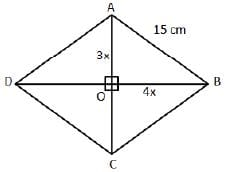In right angle triangle AOB,

AB2 = AO2 + BO2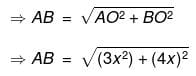=>15 = 5x

=>x = 3cm.

Therefore, we can say that the length of diagonals = 6x and 8x or 18 and 24 cm.

Hence, the area of the rhombus = 1/2 * 18 * 24 = 216 cm2. Therefore, option E is the correct answer.

Test: XAT Quant 2018 - Question 4

The price of a product is P. A shopkeeper raises its price by X% and then offers a discount of Y% on the raised price. The discounted price again becomes P. If Y is the difference between X and Y, then find X.

Detailed Solution for Test: XAT Quant 2018 - Question 4
Let the cost price of the article be Rs.100.

The shopkeeper raises the price by x% and then decreases it by y%.

As a result, he reaches the cost price of the article.

Also, it has been given that y is the difference between y% and x%.

y = x — y

2y = x

We know that (1 + 2y)(1 - y) * 100 = 100

(1 + 2y)(1 - y) = 1

1 - y + 2y - 2y2 = 1

2y2 - y = 0

2y = 1

y = 1/2 or 0.5

x = 2y

=> x = 1 or x = 100%

Therefore, option D is the right answer.

Test: XAT Quant 2018 - Question 5

A mixture comprises water and liquids A and B. The volume of water is 1/3rdof the total mixture and the volume of liquids A and B are in the ratio 5:3. To remove the water, the mixture is passed through a porous medium which completely absorbs the water and partially absorbs liquid A. Altogether this porous medium absorbs 200 ml of the initial mixture. If the ratio of volume of liquids A and B in the residual concentrated mixture becomes 7:9 then find the volume of water absorbed by the porous medium.

Detailed Solution for Test: XAT Quant 2018 - Question 5
Liquids A and B are in the ratio 5:3. The volume of water is one-third the total mixture.

Let us assume the volume of the total mixture to be 24x.

Volume of liquid A = 10x

Volume of liquid B = 6x

Volume of water = 8x

The mixture is passed through some medium that absorbs water completely and some quantity of liquid A.

Water absorbed = 8x

Let the amount of liquid A absorbed be y.

8x+y = 200

=> y = 200-8x —(1)

It has been given that (10x-y)/6x = 7/9

Substituting (1), we get,

(10x – 200+8x)/6x = 7/9

(18x-200)/6x = 7/9

162x – 1800=42x

120x = 1800

=> x = 1800/120

Amount of water absorbed = 8*1800/120 = 120 ml.

Therefore, option E is the right answer.

Test: XAT Quant 2018 - Question 6

A coin of radius 3 cm is randomly dropped on a square floor full of square shaped tiles of side 10 cm each. What is the probability that the coin will land completely within a tile? In other words, the coin should not cross the edge of any tile.

Detailed Solution for Test: XAT Quant 2018 - Question 6
The radius of the coin is 3 cm.

So, if the coin should not cross the edge, the centre of the coin should at least be 3 cm away from the edge of the tile.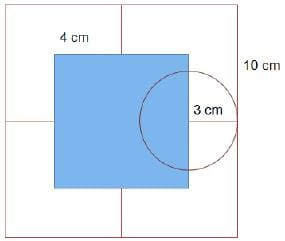In the given diagram the center of the circle should lie in the blue region. As we can see, the area in which the centre of the coin can fall is a square of side 10-3-3 = 4 cm.

Therefore, the area in which the centre of the coin can fall is 16 square cm.

Area of the tile = 100 square cm.

Required probability = 16/100 = 0.16.

Therefore, option E is the right answer.

Test: XAT Quant 2018 - Question 7

It takes 2 liters to paint the surface of a solid sphere. If this solid sphere is sliced into 4 identical pieces, how many liters will be required to paint all the surfaces of these 4 pieces.

Detailed Solution for Test: XAT Quant 2018 - Question 7
2 litres are required to paint the surface of a solid sphere.

Surface area of the solid sphere = 4π * r2

Now, the solid sphere is cut into 4 identical parts. This is possible only when the sphere is cut into 4 quarter spheres.

After making the first cut, 2 hemispheres will be formed. 2 circles of area π * r2 will get exposed in addition to the surface area of the sphere (the base surface of the bottom hemisphere and the base of the top hemisphere).

Now, another perpendicular cut will be made along the diameter of the sphere. 2 additional surfaces will get exposed again (one on left hemisphereand theanother on the right hemisphere).

Area exposed after making 4 identical pieces = 4* π * r2 + 2 * π * r2 + 2* π * r2

=8 * π * r2

2 litres of paint is required to paint an area of 4π * r2

=> 4 litres of paint will be required to paint an area of 8 * π * r2.

Therefore, option D is the right answer.

Test: XAT Quant 2018 - Question 8

Every day a person walks at a constant speed, V1 for 30 minutes. On a particular day, after walking for 10 minutes at V1, he rested for 5 minutes. He finished the remaining distance of his regular walk at a constant speed, V2, in another 30 minutes. On that day, find the ratio of V2 and his average speed (i.e., total distance covered /total time taken including resting time).

Detailed Solution for Test: XAT Quant 2018 - Question 8
The man walks with a speed of V1 for 30 minutes.

=> Distance covered = 30*V1.

On a particular day, he walks for 10 minutes at V1, takes a rest of 5 minutes, and then covers the distance by walking at V2 for 30 minutes.

=> 10*V1 + 30*V2 = 30*V1

20*V1 = 30*V2

V1 = 1.5V2 …… (1)

=> Total distance = 30*1.5*V2 = 45V2.

Now, we know that the person took 10 + 5 + 30 = 45 minutes to cover the entire distance.

=> Average speed = 45V2/45 = V2.

Ratio of V2 and the average speed = 1:1.

Therefore, option A is the right answer.

Test: XAT Quant 2018 - Question 9

A boat, stationed at the North of a lighthouse, is making an angle of 30° with the top of the lighthouse. Simultaneously, another boat, stationed at the East of the same lighthouse, is making an angle of 45° with the top of the lighthouse. What will be the shortest distance between these two boats? The height of the lighthouse is 300 feet. Assume both the boats are of negligible dimensions.

Detailed Solution for Test: XAT Quant 2018 - Question 9
One boat is stationed to the North of the light house and the other boat is stationed to the East of the light house.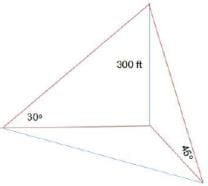The boat stationed to the East subtends an angle of 45 degrees and the boat stationed to the North subtends an angle of 30 degrees. Now, distance between the boat stationed to the East and the light house, d1 = tap 45

300/ d1 = 1

=> d1 = 300 feet

Distance between the boat stationed to the North and the light house,d2 = tan 30

300/d2 = 1/√3

=>d2 = 300√3

Shortest distance between the 2 boats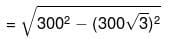= 300 * √4

=600 feet

Therefore, option C is the right answer.

Test: XAT Quant 2018 - Question 10

Two different quadratic equations have a common root. Let the three unique roots of the two equations be A, B and C - all of them are positive integers. If (A + B + C) = 41 and the product of the roots of one of the equations is 35, which of the following options is definitely correct?

Detailed Solution for Test: XAT Quant 2018 - Question 10
It has been given that A+B+C = 41.

Let the common root be B.

All the roots are positive integers.

The product of the roots of one of the equations is 35.

35 can be obtained only in 2 ways - either as 5*7 or 35*1.

A+B+C = 41.

If A and B are 5 and 7 in any order, then C = 41 - 5 - 7 = 29.

If A and B are 35 and 1 in any order, then C = 41 - 35 -1 =5.

As we can see, in either case, 5 is one of the 3 roots.

Therefore, option C is the right answer.

Test: XAT Quant 2018 - Question 11

An antique store has a collection of eight clocks. At a particular moment, the displayed times on seven of the eight clocks were as follows: 1:55 pm, 2:03 pm, 2:11 pm, 2:24 pm, 2:45 pm, 3:19 pm and 4:14 pm. If the displayed times of all eight clocks form a mathematical series, then what was the displayed time on the remaining clock?

Detailed Solution for Test: XAT Quant 2018 - Question 11
Let us find out the difference between the times given to figure out the pattern.

The times given are 1:55 pm, 2:03 pm, 2:11 pm, 2:24 pm, 2:45 pm, 3:19 pm and 4:14 pm.

The difference between 2 consecutive times given are 8 minutes, 8 minutes, 13 minutes, 21 minutes, 34 minutes, and 55 minutes.

We can observe that the differences between the times are in the Fibonacci series.

8 + 13 = 21

21+13 = 34

34 + 21 = 55

The Fibonacci series is as follows:

1,1,2,3,5,8,13,21,34,55.

But the first difference in the times given is 8.

Therefore, the missing time must be such that it divides the interval of 8 minutes into 3 minutes and 5 minutes.

The missing time should be 1:58 pm and hence, option B is the right answer.

Test: XAT Quant 2018 - Question 12

The number of boys in a school was 30 more than the number of girls. Subsequently, a few more girls joined the same school. Consequently, the ratio of boys and girls became 3:5. Find the minimum number of girls, who joined subsequently.

Detailed Solution for Test: XAT Quant 2018 - Question 12
Let the number of girls in the school be G.

=> Number of boys = G+30.

Some girls joined the class and the number of boys and girls became 3:5.

Let the number of girls who joined the class be 'X'.

It has been given that (G+30)/ (G+X) = 3/5

5G + 150 = 3G + 3X

2G + 150 = 3X

=> X = (2G/3) + 50.

2G has to be divisible by 3.

Therefore, the least value that G can take is 3.

When G = 3, X = 2 + 50

X = 52.

The least number of girls who could have joined is 52.

Therefore, option E is the right answer.

Test: XAT Quant 2018 - Question 13

A girl travels along a straight line, from point A to B at a constant speed, V1 meters/sec for T seconds. Next, she travels from point B to C along a straight line, at a constant speed of V2 meters/sec for another T seconds. BC makes an angle 105° with AB. If CA makes an angle 30° with BC, how much time will she take to travel back from point C to A at a constant speed of Vj meters/sec, if she travels along a straight line from C to A?

Detailed Solution for Test: XAT Quant 2018 - Question 13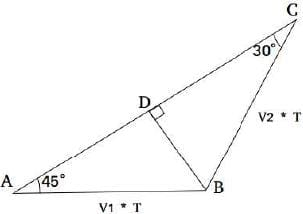We draw BD perpendicular to AC.

In right angled triangle BDC, BD / BC = sin 30°

or, BD = (V2 * T)/2 ……. (i)

In right angled triangle BDA, BD / BA = sin 45°

Or, BD = (V1 * T)/a/2 …… (ii)

From (i) and (ii),we get

V2/V1 = √2

Total distance to be travelled from C to A = CD + DA = √3 BD + BD

= BD(1 +√3 )

Replacing BD = (V2 * T)/2 in the above equation,

CA = (V2 * T) /2(1 +√3 )

Time taken at speed V2 = 0.5(√3 + 1)T

Hence, option C is the correctanswer.

Test: XAT Quant 2018 - Question 14

In the final semester, an engineering college offers three elective courses and one mandatory course. A student has to register for exactly three courses: two electives and the mandatory course. The registration in three of the four courses is: 45, 55 and 70. What will be the number of students in the elective with the lowest registration?

Detailed Solution for Test: XAT Quant 2018 - Question 14
The number of candidates registered for 3 of the 4 courses is 45, 55, and 70.

There are 2 cases.

70 can be the total number of students registered in an elective or the mandatory course.

Case (i):

70 students have registered in an elective.

Each student will choose 2 elective.

=> Total number of electives chosen = 2* total number of students.

45+55+70 = 2*number of students.

Number of students = 170/2 = 85.

In this case, at least 45 students would have selected an elective.

Case (ii):

70 students have registered in the mandatory class.

=> 45+55+x = 2*70

=> x =40.

As we can see, the number of students registered in an elective can be 40 or 45. Therefore, option E is the right answer.

Test: XAT Quant 2018 - Question 15

X and Y are the digits at the unit's place of the numbers (408X) and (789Y) where X ≠ Y. However, the digits at the unit's place of the numbers (408X) 63 and (789Y) 85 are the same. What will be the possible value(s) of (X + Y)?

Detailed Solution for Test: XAT Quant 2018 - Question 15
All numbers from 1 to 9 repeat their last digits over a cycle of 4.

63 can be written as 4k+3.

85 can be written as 4k+1.

Some number's third power should yield the first digit as some number's first power.

23 will yield 8 as the last digit (2 and 8 is a possible solution).X+Y = 10

33 will yield 7 as the last digit (3 and 7 is a possible solution).X + Y = 10

43 will yield 4 as the last digit and hence, can be eliminated.

5 and 6 yield 5 and 6 respectively as the last digit for any power and hence, can be eliminated.

73 will yield 3 as the last digit (7 and 3 is a possible solution). X+Y=10.

83 will yield 2 as the last digit. 8+2 =10.

As we can see, X+Y = 10 in all the cases. Therefore, option B is the right answer.

Test: XAT Quant 2018 - Question 16

If 2 ≤ |x -1| x |y + 3| ≤ 5 and both x and y are negative integers, find the number of possible combinations of x and y.

Detailed Solution for Test: XAT Quant 2018 - Question 16
2 ≤ |x -11 x |y + 3| ≤ 5

The product of two positive number lies between 2 and 5.

As x is a negative integer, the minimum value of |x -1| will be 2 and the maximum value of |x -1| will be 5 as per the question.

When, |x -1| = 2, |y + 3| can be either 1 or 2.

So, for x = -1, y can be - 4 or - 2 or - 5 or -1

Thus, we get 4 pairs of (x, y)

When |x -1|= 3, |y + 3| can be 1 only

So, for x = - 2, y can be -4 or -2

Thus, we get 2 pairs of the values of (x, y)

When |x -1| = 4, |y + 3| can be 1 only So, for x = - 3, y can be Apr -2 Thus, we get 2 pairs of the values of (x, y) When |x -1| = 5, |y + 3| can be 1 only

So, for x = - 4, y can be -4 or -2

Thus, we get 2 pairs of the values of (x, y)

Therefore, we get a total of 10 pairs of the values of (x, y)

Hence, option E is the correct answer.

Test: XAT Quant 2018 - Question 17

David has an interesting habit of spending money. He spends exactly £X on the Xthday of a month. For example, he spends exactly £5 on the 5th of any month. On a few days in a year, David noticed that his cumulative spending during the last 'four consecutive days' can be expressed as 2N where N is a natural number. What can be the possible value(s) of N?

Detailed Solution for Test: XAT Quant 2018 - Question 17
The sum of 4 consecutive numbers should be a power of 2.

The powers of 2 are 1, 2, 4, 8, 16, 32, and 64,128. The maximum possible value that 4 consecutive days can take is 28 + 29 + 30 + 31 = 118.

We can eliminate 1, 2, 4, and 8 since sum of 4 consecutive integers is always greater than 9.

Let the first day be x.

x+x+1+x+2+X+3=16

=> 4x = 10 x = 2.5

we can eliminate 16.

Let us check for 32.

x+x+1+X+2+x+3 = 32

4x=26

x is not an integer.

Let us check the case in which x is the last day of the month.

Even in the month of February, the least value that x can take is 28.

28+1+2+3 = 34 >32.

We can eliminate 32 as well.

Let us check for 64.

4x+6 = 64

4x = 58

The sum of no 4 consecutive days in the same month can be expressed as 4k+6.

Let us check the cases in which 2 months are involved.

29+30+1+2 = 62 < 64.

30 + 31 +1 + 2 = 64. This is a possible combination.

There are 7 months with 31 days in a year. We have to eliminate December since 1 will spill over to the 1st of January of the next year.

Therefore, in a year, there will be 6 such instances. Therefore, option B is the right answer.

Test: XAT Quant 2018 - Question 18

A cone of radius 4 cm with a slant height of 12 cm was sliced horizontally, resulting into a smaller cone (upper portion) and a frustum (lower portion). If the ratio of the curved surface area of the upper smaller cone and the lower frustum is 1:2, what will be the slant height of the frustum?

Detailed Solution for Test: XAT Quant 2018 - Question 18

The ratio of the curved surface area of the upper cone to the lower frustum- is 1:2.

=> the ratio of the curved surface area of the upper cone to the total cone = 1:3.

Curved surface area (CSA) of a cone = π* r * l

For the given cone, the slant height, l = 12cm

CSA of the cone = 48 * π

CSA of the smaller cone = 16 * π

Both the slant height and the radius would have been reduced by the same ratio. Let that ratio be x.

x2 * 48 * π = 16π

Slant height of the smaller cone = 12√3

Slant height of the frustum = 12 -12√3

= 12 * √3 – 1/√3

= 12 * (3-√3)/3

=12-4√3

Therefore, option D is the right answer.

Test: XAT Quant 2018 - Question 19

Two circles with radius 2R and √2R intersect each other at points A and B. The centers of both the circles are on the same side of AB. 0 is the center of the bigger circle and AOB is 60°. Find the area of the common region between two circles.

Detailed Solution for Test: XAT Quant 2018 - Question 19
Let us draw the diagram according to the given info,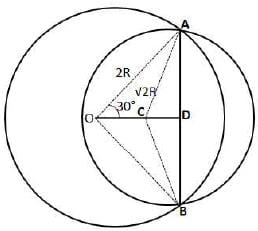We can see that AD = A0*cos60° = 2R* 1/2 = R

In triangle, ACD

=>sinACD = AC/AC

=>sinACD = R/√2 * R = 1/√2

=>∠ACD = 45°

By symmetry we can say that ∠BCD = 45°

Therefore we can say that ∠ACB = 90°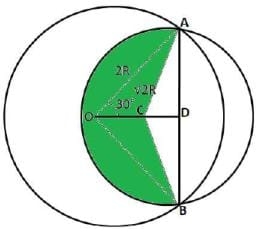Hence, the area colored by green color = 270°/360° * π * (√2R)2 = 3/2 * π * R2... (1)

Area of triangle ACB = 1/2 * R* 2R = R2... (2)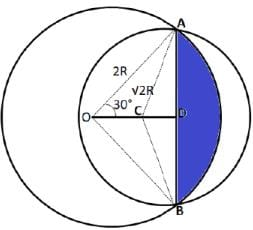Area shown in blue color = 60°/360° *π* (2R)2-√3/4 * (2R)2 = 2/3 *π* R2-√3 * R2... (3)

By adding (1) + (2) + (3)

Therefore, the area of the common region between two circles = 3/2 * π * R2 + R2 + 2/3 * π * R2 - √3 * R2

=> (13π/6 + 1 +√3)R2Hence, option C is the correct answer.

Test: XAT Quant 2018 - Question 20

These statements provide data that may help answer the respective questions. Read the questions and the statements and determine if the data provided by the statements is sufficient or insufficient, on their own or together, to answer the questions. Accordingly, choose the appropriate option given below the questions.

A group of six friends noticed that the sum of their ages is the square of a prime number. What is the average age of the group?

Statement I: All members are between 50 and 85 years of age.

Statement II: The standard deviation of their ages is 4.6.

Detailed Solution for Test: XAT Quant 2018 - Question 20
Sum of the ages of the 6 friends is the square of a prime number.

Statement I states that all the members were aged between 50 and 85 years. There are 6 friends in total. Therefore, the sum of the ages can vary from 6*50 to 6*85.

Sum of the ages of the 6 persons should lie between 300 and 510.

361 is the only square of a prime number (19) in the given range. Therefore, statement I alone is sufficient to answer the question.

Statement II states that the standard deviation of their ages is 4.6. SD gives us how much the values deviate from the mean. We cannot obtain the mean using the SD. Therefore, statement II alone is insufficient to answer the question without knowing the values in the data set.

Therefore, option A is the right answer.

Test: XAT Quant 2018 - Question 21

These statements provide data that may help answer the respective questions. Read the questions and the statements and determine if the data provided by the statements is sufficient or insufficient, on their own or together, to answer the questions.Accordingly, choose the appropriate option given below the questions.

Harry and Sunny have randomly picked 5 cards each from a pack of 10 cards, numbered from 1 to 10. Who has randomly picked the card with number 2 written on it?

Statement I: Sum of the numbers on the cards picked by Harry is 5 more than that of Sunny.

Statement II: One has exactly four even numbered cards while the other has exactly four odd numbered cards.

Detailed Solution for Test: XAT Quant 2018 - Question 21
Harry and Sunny have cards numbered from 1 to 10,

Sum of the number of cards picked by them = 1 +2+3+...+10 = 55.

Sum of the numbers on the cards picked by Harry is 5 more than that of Sunny.

Let x be the sum of the numbers picked by Sunny.

x+x+5 = 55

x = 25

25 can be written as 10+9+1 +2+3 or 9+7+5+1 +3 etc. (There are many such combinations). We cannot determine the person who has card number 2.

Statement I alone is insufficient.

Statement II states that one of the 2 persons has exactly 4 even-numbered cards.

The person with 4 even-numbered cards might or might not contain card number 2. Therefore, statement II alone is insufficient.

Combining both the statements, we know that the sum of the numbers on the cards with Harry is 5 more than the sum of the number on the cards with Sunny. Therefore, the sum of the number of cards with Sunny should be 25 and Harry should be 30.

Sum of the 5 even numbers = 2+4+6+8+10 = 30.

Sum of the 5 odd numbers = 1+3+5+7+9 = 25.

By replacing one of the odd numbers with even numbers, we have to make the sums 30 and 25. We cannot replace an odd number with an even number and still get the sum as 25. Therefore, the set with 4 odd numbers must add up to 30 and the set with 4 even numbers must add up to 25. We can interchange 1 with 6 or 3 with 8 or 5 with 10. The card with number 2 will always remain with the person with 4 even cards. The card with number 25 will always remain with Sunny. Therefore, we can determine the answer using both the statements together and hence, option D is the right answer.

Test: XAT Quant 2018 - Question 22

Instructions

Six teams are playing in a hockey tournament where each team is playing against every other team exactly once. At an intermediate stage, the status is as follows: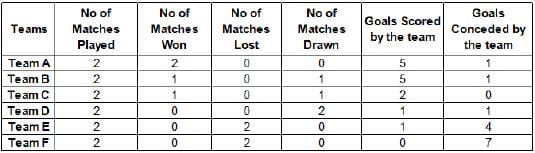Notes:

The team that scores more goals than it concedes wins the match, while if both the teams score the same no. of goals, the match is declared drawn.

In a match played between Team X and Team Y, if team X scores 1 and concedes none, then the score line would read: Team X - Team Y (1-0)

Q. Which of the following matches are yet to be played?

Test: XAT Quant 2018 - Question 23

Instructions

Six teams are playing in a hockey tournament where each team is playing against every other team exactly once. At an intermediate stage, the status is as follows:Notes:

The team that scores more goals than it concedes wins the match, while if both the teams score the same no. of goals, the match is declared drawn.

In a match played between Team X and Team Y, if team X scores 1 and concedes none, then the score line would read: Team X - Team Y (1-0)

Q. Which of the following score line is a possible outcome in the tournament?

Detailed Solution for Test: XAT Quant 2018 - Question 23

Test: XAT Quant 2018 - Question 24

Instructions

Six teams are playing in a hockey tournament where each team is playing against every other team exactly once. At an intermediate stage, the status is as follows:Notes:

The team that scores more goals than it concedes wins the match, while if both the teams score the same no. of goals, the match is declared drawn.

In a match played between Team X and Team Y, if team X scores 1 and concedes none, then the score line would read: Team X - Team Y (1-0)

Q. Which of the following score line is not a possible outcome in the tournament?

Detailed Solution for Test: XAT Quant 2018 - Question 24

Test: XAT Quant 2018 - Question 25

Instructions:The graph below represent the performance of four professors, across years, measured on four variables: Percentage of time spent on research, feedback on (a scale of 10. right-hand side) and number of publications (right-hand side). Assume that the cumulative time spent per year on research and teaching activities are sane for all four professors and each of them taught only one course of 90 classroom hours.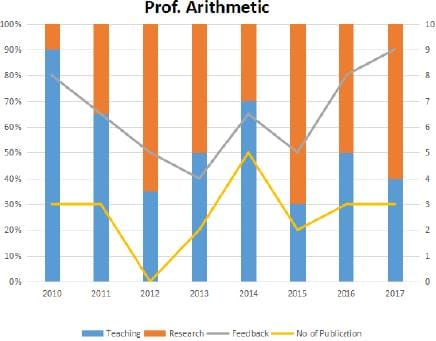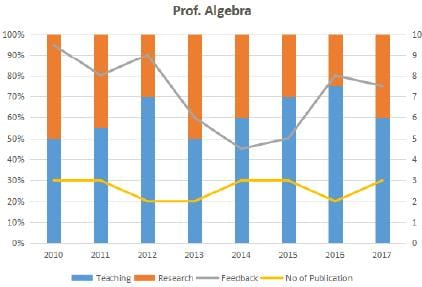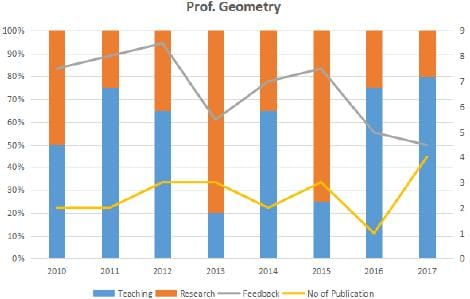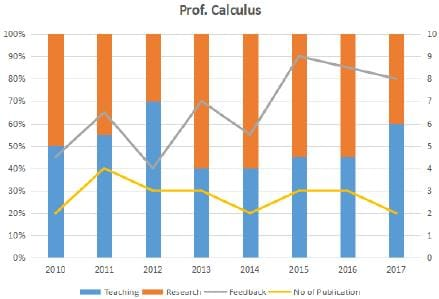Q. Which of the following shows the maximum year to year percentage growth in feedback?

Detailed Solution for Test: XAT Quant 2018 - Question 25

Test: XAT Quant 2018 - Question 26

Instructions:The graph below represent the performance of four professors, across years, measured on four variables: Percentage of time spent on research, feedback on (a scale of 10. right-hand side) and number of publications (right-hand side). Assume that the cumulative time spent per year on research and teaching activities are sane for all four professors and each of them taught only one course of 90 classroom hours.Q. Count the number of instances in which "annual decreasing efforts in research" is accompanied with "annual increase in feedback"?

Detailed Solution for Test: XAT Quant 2018 - Question 26

Test: XAT Quant 2018 - Question 27

Instructions:The graph below represent the performance of four professors, across years, measured on four variables: Percentage of time spent on research, feedback on (a scale of 10. right-hand side) and number of publications (right-hand side). Assume that the cumulative time spent per year on research and teaching activities are sane for all four professors and each of them taught only one course of 90 classroom hours.Research efficiency is the ratio of cumulative number of publication for a period of 3years to the cumulative number of hours spent on research activity in those 3 years.

Q. Which of the following professors is the least efficient researcher for the period 2015 to 2017?

Information about Test: XAT Quant 2018 Page
In this test you can find the Exam questions for Test: XAT Quant 2018 solved & explained in the simplest way possible. Besides giving Questions and answers for Test: XAT Quant 2018, EduRev gives you an ample number of Online tests for practice

### How to Prepare for CAT

Read our guide to prepare for CAT which is created by Toppers & the best Teachers